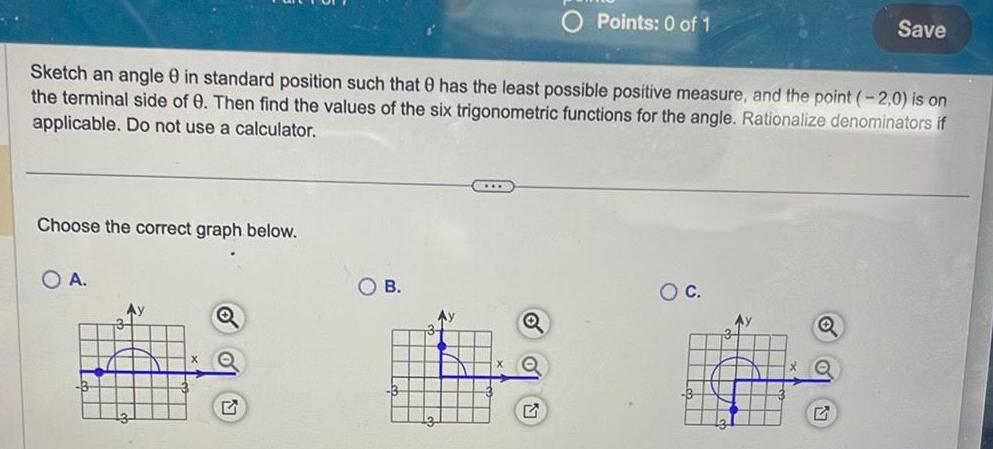Question:

# Choose the correct graph below Sketch an angle 0 in standard

Last updated: 9/14/2023Choose the correct graph below Sketch an angle 0 in standard position such that 8 has the least possible positive measure and the point 2 0 is on the terminal side of 0 Then find the values of the six trigonometric functions for the angle Rationalize denominators if applicable Do not use a calculator O A Q Points 0 of 1 B O C H Save 17